Maths-
General
Easy

Question

# Graph the following functions by filling out the X/Y chart using the given inputs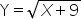X -9 -8 -5 0 7 YHint:

## The correct answer is: 0,1,2,3,4

### Step by step solution:The given equation isPutting x = -9 in the above equation, we get the value of y as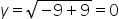Similarly, putting x = -8, we get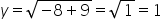Putting x = -5 we have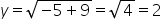Putting x = 0 we have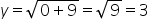Finally, putting x = 7, we have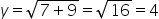Completing the table, we have x -9 -8 -5 0 7 y 0 1 2 3 4 We plot these points on the xy plane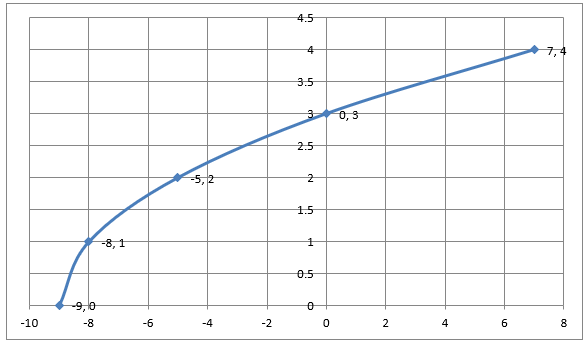After plotting the points, we join them with a smooth curve to get the graph of the equation.

We can find the tabular values for any points of x and then plot them on the graph. But we usually choose values for which calculating y is easier. Either way, we need points satisfying the equation to plot its graph.#### With Turito Foundation.#### Get an Expert Advice From Turito.